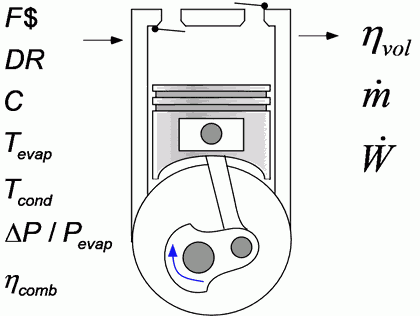Contents - index

Compressor3_CLProcedure Compressor3_CL is a model of a compressor representative of the reciprocating compressors used for refrigeration and air conditioning applications.  The model, which is based on method described by Jahnig, Reindl, and Klein, ASHRAE Transactions, Vol 106, Pt.2, 2000, determines the volumetric efficiency, mass flow rate and power.

Inputs:

F\$:  refrigerant

DR:  displacement rate  (m^3/s, ft^3/hr)

C:  clearance volume ratio (-)  (C normally ranges between 0.02 and 0.035.  A value of 0.025 is recommended if other information is not available.)

T_evap:  evaporating temperature (C, K, F, R)

DELTAT_sh:  degrees of superheat at compressor inlet (DELTAK, DELTAF)

T_cond:  condensing temperature  (C, K, F, R)

dP_evap:  pressure loss in the intake valves divided by the pressure in the evaporator (-).  (This parameter normally ranges between 0.05 and 0.25.  A value of 0.1 is recommended.)

eta_cm:  combined efficiency of the compressor and motor.  (The value normally ranges between 0.5 and 0.7.  If a negative value is supplied, a default correlation determined from experimental data will be used.)

Outputs:

eta_vol:  volumetric efficiency

m_dot: mass flow rate of refrigerant (kg/s, lbm/hr)

W_dot:   compressor power (W)

h_out: specific enthalpy at compressor exit assuming adiabatic operation (J/kg, kJ/kg, Btu/lbm)

Example:

\$UnitSystem SI C kPa mass kJ

F\$='R134a'

DR=0.345 [in^3]*convert(in^3,m^3)*3550 [1/min]*convert(1/min, 1/sec)

C=0.025

DELTAT_sh=5 [DELTAK]

T_evap=-25 [C]

T_cond=40 [C]

dP_evap=0.1

eta_cm=0.55

Call compressor3_cl(F\$, DR, C, T_evap, DELTAT_sh, T_cond, dP_evap, eta_cm: eta_vol, m_dot, W_dot, h_out)

{Solution:

eta_vol=0.7256 [-]

h_out=334.3 [kJ/kg]

m_dot=0.001306 [kg/s]

W_dot=0.124 [kW]

}